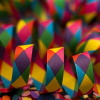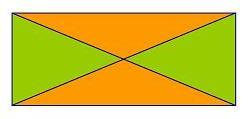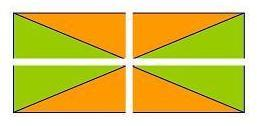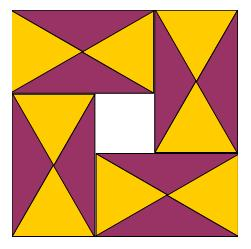You may also likeRationals Between...

What fractions can you find between the square roots of 65 and 67?Repetitiously

Can you express every recurring decimal as a fraction?Equal Equilateral Triangles

Can you make a regular hexagon from yellow triangles the same size as a regular hexagon made from green triangles ?

The Square Hole

Age 14 to 16Challenge Level

Incidentally, did you notice that the yellow and purple triangles have the same area ? This doesn't require the particular case of one triangle being equilateral, any rectangle split into 4 areas by its diagonals will do.More obvious now ?Anyway back to the area of the Square Hole :Thank-you to Clem, and to Marta & Brittany from MaST Community Charter School, and others who sent in solutions.

Seeing the image as a 'hole' surrounded by four rectangles, with each rectangle made from $2$ yellow (equilateral) and $2$ purple triangles.

The 'height' of the equilateral triangles is $\sqrt{3}$ divided by 2

So the dimensions of each rectangle are $1$ and $\sqrt{3}$

The side of the square hole is therefore $\sqrt{3} - 1$